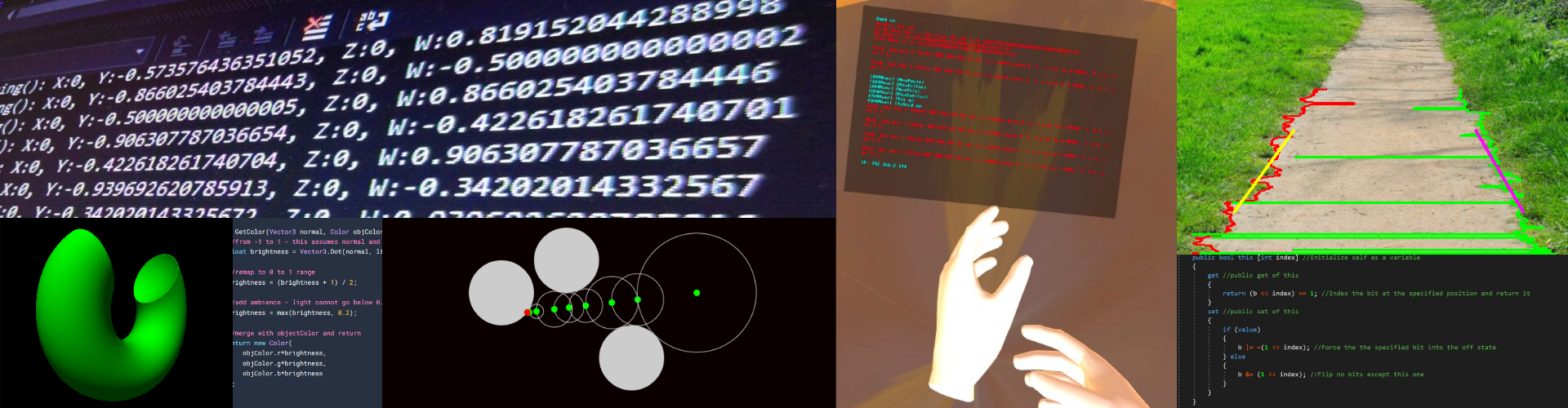This is a turtle project I built which is essentially an advanced logo. When you start it, type help and it will give you a list of commands. This was my first attempt at a programming language, my next being Javelin. Copy the program into your python IDE to run it. If you are wondering why I called it breadcode, it is because I ate bread for breakfast!

```print('initializing breadcode...')
r = 0
repeat = 0
items = []
var = {}
import turtle as x
t = x.Turtle()
l = x.Turtle()
l.ht()
l.pu()
x.mode('logo')
t.speed('fastest')
x.title('Breadcode - a turtle program by John Williams')
x.screensize(5000,5000)
#FUNCS
def num(num):
try:
n = int(num)
return True
except:
return False
def run_repeat(l, times):
global repeat
print('Click the Breadcode window and press z to abort')
for i in range(int(times)):
for cmd in range(len(l)):
run(l[cmd])
if repeat == 0:
break
x.listen()
if repeat == 0:
break
def character(string,char):
for letter in range(len(string)):
if string[letter] == char:
pos = letter
return pos
def abort():
global repeat
repeat = 0
def run(cmd):
global repeat
global r
cmd = cmd.replace(' ', '')
cmd = cmd.upper()
try:
if cmd == 'HELP':
print('Commands:\n\tsfill: starts the fill\n\tefill: ends the fill\n\tcol=XXX: takes a colour from XXX for the fill\n\thcol=XXX: takes a hexadecimal colour from XXX for the fill pen\n\tpen=XXX: sets the pen mode where XXX is u or d (up/down)\n\tsize=XXX: sets the size of the pen where XXX is an integer\n\td=XXX: sets the direction of the pen where XXX is an integer\n\tbg=XXX sets the background where XXX is a colour\n\trepeatXXX: repeat what is indented (1 space) XXX times\t\tv0.1\n\tVariable assingment and maths is allowed\t\t\tv0.3\n\n\tfXXX:,bXXX,lXXX,rXXX: Move the pen forwards,backwards,left and right XXX pixels or degrees respectively\n\n\tdel all: clear the canvas\n\tundo: undoes the last movement THERE IS NO RESPECTIVE REDO\n\tcoordsX,Y: sets the coordinates of the pen as X,Y\n\tgetallvars: prints all the variables you have set up\n\nBreadcode is not case sensitve')
elif cmd == 'SFILL':
t.begin_fill()
elif cmd == 'EFILL':
t.end_fill()
elif cmd[:5] == 'HCOL=':
x.colormode(255)
rgb=cmd[5:].split(',')
t.color(int(r),int(g),int(b))
elif cmd == 'PEN=U':
t.penup()
elif cmd == 'PEN=D':
t.pendown()
elif cmd[:5] == 'SIZE=':
if cmd[1:] in var:
t.pensize(int(var[cmd[1:]]))
else:
t.pensize(int(cmd[5:]))
elif cmd[:2] == 'D=':
elif cmd[:4] == 'COL=':
if num((cmd[4:]).replace(',', '')):
RGB = cmd[4:].split(',')
x.colormode(255)
t.color(int(RGB), int(RGB), int(RGB))
else:
x.colormode(1.0)
t.color(cmd[4:])
elif cmd[:3] == 'BG=':
t.bgcolor(cmd[3:])
elif cmd[:6] == 'REPEAT':
repeat = 1
r = cmd[6:]
elif '=' in cmd:
pos = character(cmd,'=')
var[cmd[:pos]] = cmd[(pos+1):]
elif cmd == 'F':
if cmd[1:] in var:
t.forward(float(var[cmd[1:]]))
else:
t.forward(float(cmd[1:]))
elif cmd == 'B':
if cmd[1:] in var:
t.backward(float(var[cmd[1:]]))
else:
t.backward(float(cmd[1:]))
elif cmd == 'R':
if cmd[1:] in var:
t.right(float(var[cmd[1:]]))
else:
t.right(float(cmd[1:]))
elif cmd == 'L':
if cmd[1:] in var:
t.left(float(var[cmd[1:]]))
else:
t.left(float(cmd[1:]))
elif cmd == 'DELALL':
if input('Do you really want to continue? (y/n): ') == 'y':
x.bgcolor('black')
t.reset()
t.color('red')
elif cmd[:6] == 'COORDS':
coords = cmd.split(',')
t.goto(int((coords)[6:]), int(coords))
elif cmd[:4] == 'GET:':
print(var[cmd[4:]])
elif cmd == 'GETALLVARS':
out = str(var).replace(':', ' =')
out = out.replace('\'', '')
print(out)
elif cmd == 'UNDO':
t.undo()
elif '+' in cmd:
pos = character(cmd,'+')
var[cmd[:pos]] = (float(var[cmd[:pos]]))+(int(cmd[(pos+1):]))
elif '-' in cmd:
pos = character(cmd,'-')
var[cmd[:pos]] = (float(var[cmd[:pos]]))-(int(cmd[(pos+1):]))
elif '*' in cmd:
pos = character(cmd,'*')
var[cmd[:pos]] = (float(var[cmd[:pos]]))*(int(cmd[(pos+1):]))
elif '/' in cmd:
pos = character(cmd,'/')
var[cmd[:pos]] = (float(var[cmd[:pos]]))/(int(cmd[(pos+1):]))
else:
print('\n    (most recent line)\n       SyntaxError: Command \'' + cmd + '\' is not defined\n')
except ValueError:
print('\n    (most recent line)\n        NumError: Enter number in num command\n')
except KeyError as e:
print('\n    (most recent line)\n        VarError: Var ' + str(e) + ' is not defined\n')
except Exception as e:
print('\n    (most recent line)\n        Error ' + str(e) + ' in object \n')
x.onkey(abort, 'z')
x.listen()
t.pendown()
t.pensize(1)
t.color('red')
x.bgcolor('black')
print('type help for a list of commands')
print('done \n \n \n \n')
while True:
x.listen()
if repeat is 1:
cmd = input('... ')
if (len(cmd)==0):
run_repeat(items, r)
items = []
repeat = 0
r = 0
elif cmd == ' ':
items.append(cmd[1:])
else:
cmd = input('>>> ')
if len(cmd) > 0:
run(cmd)
```

1.Mr James says: